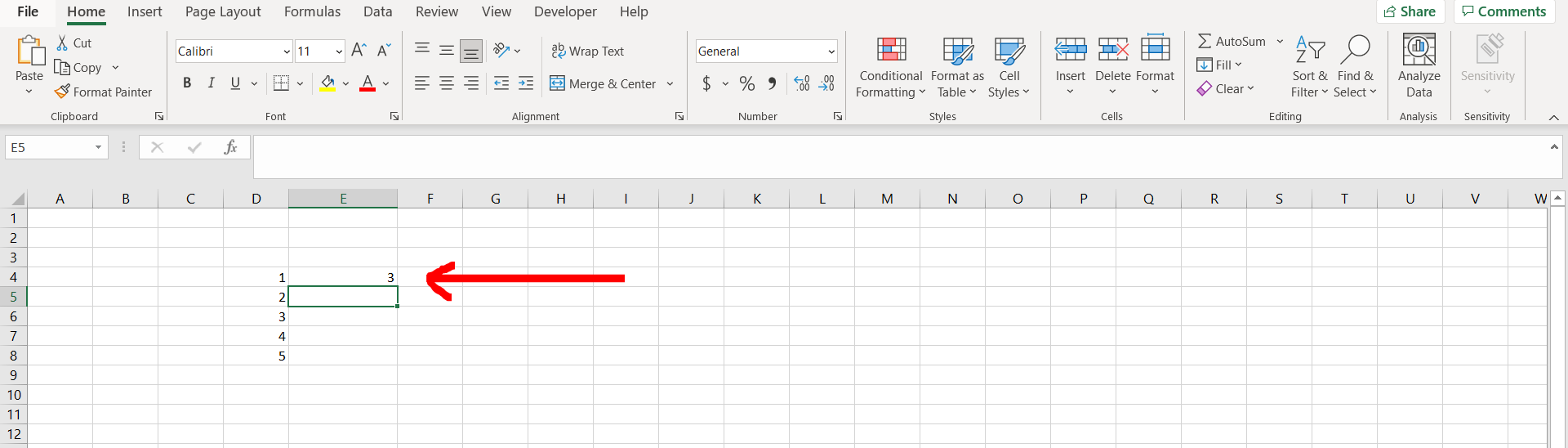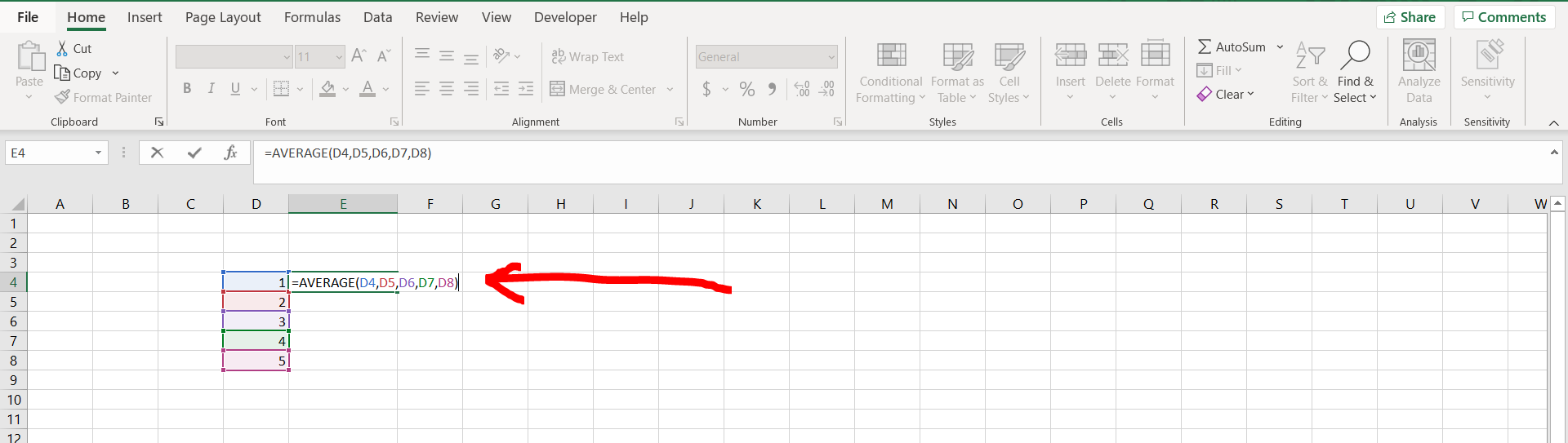# How to add cells in Excel formulaExcel offers a very interesting way to add cells in formulas. We can cater to this problem statement by toggling to multiple cells. We can perform the below mentioned way to add cells in excel formula:

### Step 1 – Excel workbook with some cells having numbers– Open the desired Excel workbook containing a text written in a formula

### Step 2 – Enter a formula– Now enter a formula and add the first cell to it. We have used the “=AVERAGE()” formula here.

### Step 3 – Add all the cells– Now after adding the first cell, add a comma (,), and reference to another cell, and then add a comma. Do this till you have entered all the cells in the formula and then hit enter.

### Step 4 – Output obtained– We can see that the formula has been used and all the cells have been added in the formula